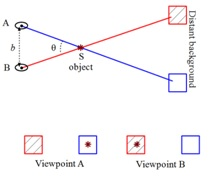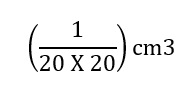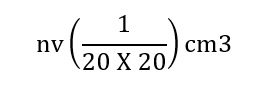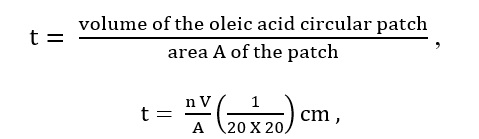# Measurement of Length:

You are already familiar with some direct methods for the measurement of length.

For example, a meter scale is used for lengths from 10-3 m to 102m.

A vernier callipers is used for lengths to an accuracy of 10-4m.

A screw gauge and a spherometer can be used to measure lengths as less as to 10-5m.

### Measurement of Large Distance:

Large distances such as the distance of a planet or a star from the earth cannot be measured directly with a metre scale. An important method in such cases is the parallax method.

A simplified illustration of the parallax of an object against a distant background due to a perspective shift is illustrated in fig. When viewed from “Viewpoint A”, the object appears to be in front of the blank square. When the viewpoint is changed to “Viewpoint B”, the object appears to have moved in front of the hatched square. This is called parallax.

The “parallax” or “the parallax angle” is the angle of inclination of the two sight-lines of the distant object. In Fig, Ɵ is the parallax angle. It is also known as the parallactic angle.

The distance between the two points of observation is called the basis. In fig, b is the basis.### Estimation of Very Small Distance:

Oleic acid is a soapy liquid with a large molecular size of the order of 10-9m. The idea is to first form a mono-molecular layer of oleic acid on the water surface. We dissolve 1 cm3 of oleic acid in alcohol to make a solution of 20 cm3. Then we take 1 cm3 of this solution and dilute it to 20 cm3, using alcohol. So, the concentration of the solution is equal toof oleic acid/cm3 of the solution. Next, we lightly sprinkle some lycopodium powder on the surface of the water in a large trough and we put one drop of this solution in the water. The oleic acid drop spreads into a thin, large and roughly circular film of molecular thickness on the water surface. Then, we quickly measure the diameter of the thin film to get its area A. Suppose we have dropped n drops in the water. Initially, we determine the approximate volume of each drop (V cm3).

Volume of n drops of solution

nV cm3

Amount of oleic acid in this solutionLet the thickness of the film is t, thenWhere V is in cm3 and A is in cm2.  If we assume that the film has a mono-molecular thickness, then this becomes the size or diameter of a molecule of oleic acid. The value of this thickness comes out to be of the order of 10-9 m.

### Range of Lengths:

The sizes of the objects we come across in the universe vary over a very wide range. These may vary from the size of the order of 10-14 m of the tiny nucleus of an atom to the size of the order of 1026 m of the extent of the observable universe. We also use certain special length units for short and large lengths. These are

UnitExpressionMathemetical Expression
1 fermi1 f10-15 m
1 angstrom1 Å10-10 m
1 astronomical unit1 AU (average distance of the Sun from the Earth)1.496 × 1011 m
1 light year1 ly9.46 × 1015 m (distance that light travels with velocity of 3 × 108 m s-1 in 1 year)
1 parsec3.08 × 1016 m (Parsec is the distance at which average radius of earth’s orbit subtends an angle of 1 arc second)